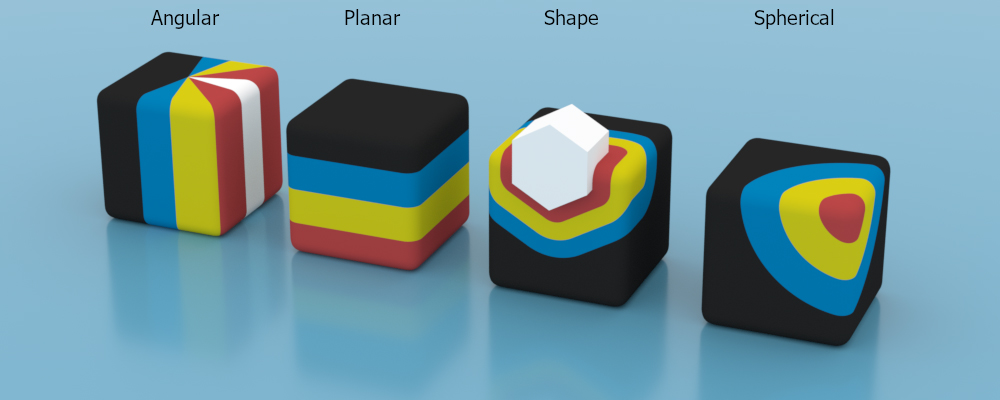# Fields

The Field nodes are essentially 3D gradients that can be used to control the output value of many different data/node types in Octane (figure 1).Figure 1: The four field types used in conjunction with a 4-color gradient

The four field types available are:

• Angular - Similar to the angular gradient but has additional options to map the angle to the gradient value
• Planar - Similar to the linear gradient but with a finite mapping
• Shape - Maps the distance between Vectron/SDF objects
• Spherical - Maps the distance of the origin in UVW space to a (clamped) gradient value